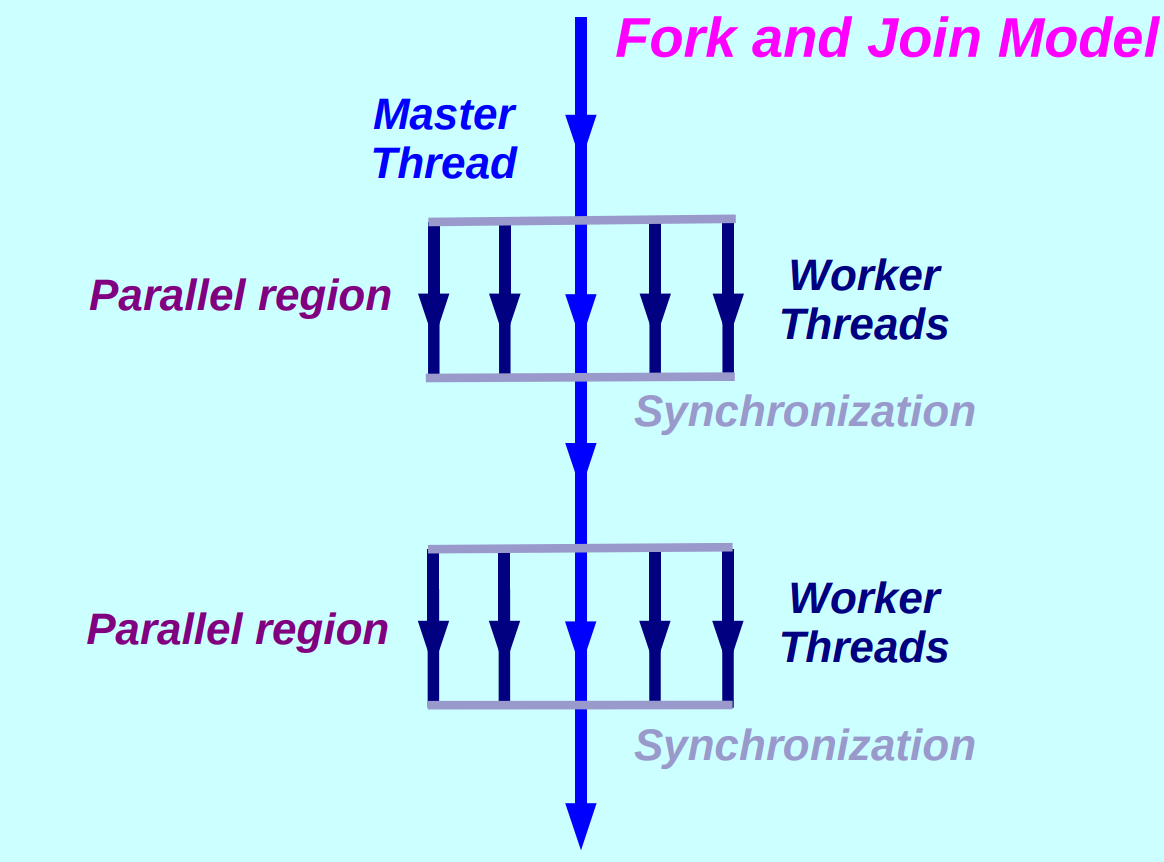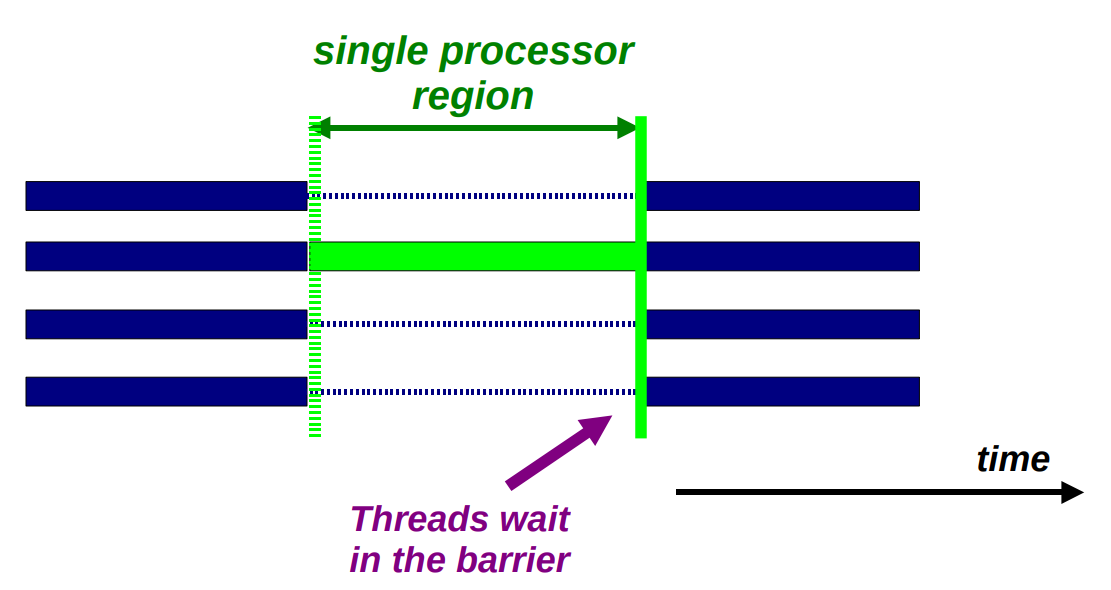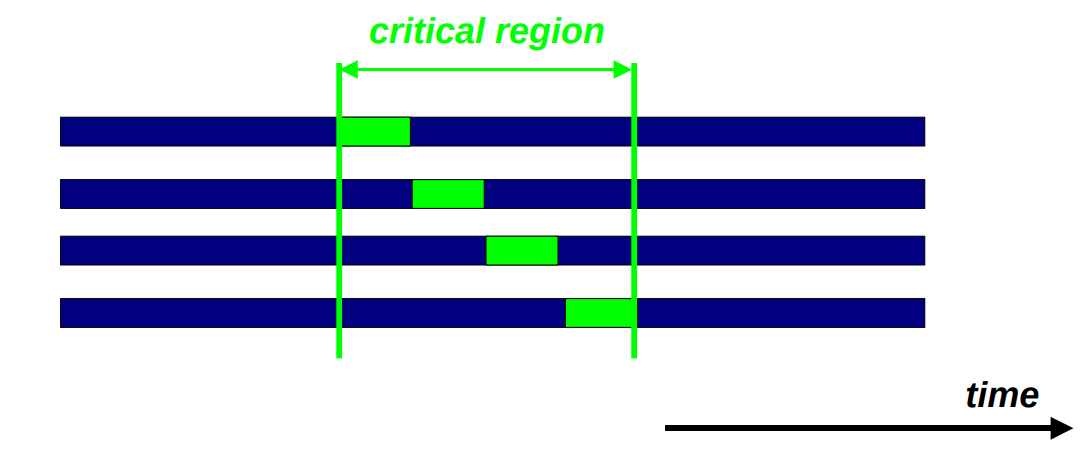## What is OpenMP?#

### OpenMP Model#

• 每个线程都有可以访问全局的共享内存。
• 数据可以是共享的也可以是私有的。
• 共享的数据可以被所有线程访问。
• 私有数据只能被拥有它的线程访问。
• 数据的传递对于编程者是透明的。
• 同步会发生，但是它大部分时候是隐式的。### Data-Sharing Attributes#

• 共享的
• 只有一个数据的实例
• 所有的线程都可以同步地读或者写，除非有特定的 OpenMP 结构保护它
• 所有数据变化都对所有线程可见

但是除非强制，并不需要变化立即可见

• 私有的
• 每一个线程持有一份数据的拷贝
• 其他线程无权访问该数据
• 数据变化只对拥有者线程可见

### The OpenMP Execution Model#

OpenMP 程序执行模型被称为 Fork and Join Model，在不需要并行执行时只有一个主线程，在需要并行时 Fork 出多个 Worker 线程，在经历了同步之后 Join 到一个主线程。## How to use OpenMP?#

### A first OpenMP example#

``````#include <stdio.h>
#include <stdlib.h>
#include <omp.h>

int main(void)
{
# pragma omp parallel for num_threads(4)
for (int i = 0; i < 20; ++i) {
printf("Hello from thread %d of %d\n", \
}
return 0;
}
``````
``````gcc -o for for.c -fopenmp
./for
``````

### Matrix example#

``````# pragma omp parallel for default(none) \
private(i, j, sum) \
shared(m, n, a, b, c)
for (i = 0; i < m; ++i) {
sum = 0.0f;
for (j = 0; j < n; ++j) {
sum += b[i][j] * c[j];
}
a[i] = sum;
}
``````

### A more elaborate example#

``````# pragma omp parallel if (n>limit) default(none) \
shared(n, a, b, c, x, y, z) private(f, i, scale)
// 使用 if 语句来避免因为矩阵过小导致的并行衰退
{
f = 1.0f;
# pragma omp for nowait
// nowait 每个线程完成自己的工作后不等待其他线程
// 而是直接执行后面的代码
for (i = 0; i < n; ++i)
z[i] = x[i] + y[i];

# pragma omp for nowait
for (i = 0; i < n; ++i)
a[i] = b[i] + c[i];

# pragma omp barrier
// 在此处显示说明要同步
// ...
scale = sum(a, 0, n) + sum(z, 0, 0) + f;
// ...
}
``````

## OpenMp in Some More Detail#

### Terminology and behavior#

• OpenMP Team := Master + Worker
• 并行域(Parallel Region)是所有线程同步执行的代码块
• 主线程 ID 永远是 0
• 线程调整（如果有的话）都只会在进入并行域之后完成
• 并行域是可以嵌套的
• if 语句可以用于并行域，如果为假则会串行执行
• 有一个 work-sharing 结构(例如 Single, Sections 等)在分配任务给 team 中的成员

### The if / private / shared clauses#

• `if (scalar expression)`
• `private(list)`
• `firstprivate(list)` 在第一个循环继承共享变量的值
• `lastprivate(list)` 根据逻辑上的最后一个线程给共享变量赋值（不是实际运行完的最后一个线程，对于 for 循环就是最后一次迭代的值）
• `shared(list)`

### The sections directive#

``````#pragma omp parallel default(none) \
shared(n,a,b,c,d) private(i)
{
#pragma omp sections nowait
// 下面两个 section 是并行的
{
#pragma omp section
for (i = 0; i < n - 1; ++i)
b[i] = (a[i] + a[i + 1] / 2);
#pragma omp section
for (i = 0; i < n; ++i)
d[i] = 1.0f / c[i];
} /*-- End of sections --*/
} /*-- End of parallel region --*/
``````

### Single processor region#### Critical Region#

``````for (i = 0; i < N; ++i) {
// ...
sum += a[i];
// ...
}
``````

``````#pragma omp critical [(name)]
````````````#pragma omp atomic [(name)]
``````

## OpenMP 3.0 supports for TASKS#

``````while(my_pointer) {
(void)do_independent_work(my_pointer);
my_pointer = my_pointer->next ;
} // End of while loop
``````

``````#pragma omp parallel
{
#pragma omp single nowait
{
while (my_pointer) {